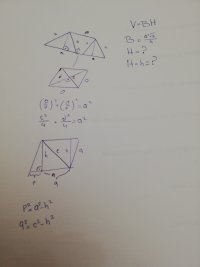# Rhombus confused

#### Loki123

##### Full Member
The base of an oblique prism is an equilateral triangle of side a, one side is normal to the base and has the shape of a rhombus with a smaller diagonal c. Calculate the volume.

After some thinking I have visualized this problem. Basically one side goes from a square to a rhombus and that's how it has 90 degrees to the base. At least that's how I understood. The answer has to include only a and c and so far the only thing I am missing is H, height of the prism, which is also h, height of the rhombus. So If I am right the question is basically, how to calculate rhombus height with a side and a smaller diagonal?

Help#### lev888

##### Elite Member
The base of an oblique prism is an equilateral triangle of side a, one side is normal to the base and has the shape of a rhombus with a smaller diagonal c. Calculate the volume.

After some thinking I have visualized this problem. Basically one side goes from a square to a rhombus and that's how it has 90 degrees to the base. At least that's how I understood. The answer has to include only a and c and so far the only thing I am missing is H, height of the prism, which is also h, height of the rhombus. So If I am right the question is basically, how to calculate rhombus height with a side and a smaller diagonal?

Help

View attachment 30684
Express the area of the rhombus 2 ways, solve the resulting equation for h.

•Loki123

#### Loki123

##### Full Member
Express the area of the rhombus 2 ways, solve the resulting equation for h.
c*d/2 = ah/2
Problem is, I don't know d or h.

#### lev888

##### Elite Member
c*d/2 = ah/2
Problem is, I don't know d or h.
Well, h is the variable in the equation, of course you don't know it.
But you can find d. What's the angle between the diagonals in a rhombus?

#### lev888

##### Elite Member
•Loki123

#### Loki123

##### Full Member
Side times height. That's what it says in the book. Is it wrong cause I got a semi-correct answer.

C^2+d^2=4a^2
D=sqrt(4a^2-c^2)

ah/2=cd/2
ah=c*sqrt(4a^2-c^2)
h=c*sqrt(4a^2-c^2)/a

V=a^2*sqrt(3)/4 * c*sqrt(4a^2-c^2)/a
V=ac*sqrt(12a^2-3c^2)/4

It's supposed to be
h=c*sqrt(4a^2-c^2)/2a
V=ac*sqrt(12a^2-3c^2)/8

#### lev888

##### Elite Member
•Loki123

#### Loki123

##### Full Member
In my book, it says
Rhombus =ah/2 =d1d2/2
I am staring at it right now.

#### lev888

##### Elite Member
In my book, it says
Rhombus =ah/2 =d1d2/2
I am staring at it right now.
d1d2/2 - sounds right. I'm assuming it's half the product of diagonals, correct?
What exactly is referenced in ah/2?

#### Loki123

##### Full Member
Rhombus side times rhombus height divided by two

#### lev888

##### Elite Member
Rhombus side times rhombus height divided by two
Please post a photo of this formula and its description.

#### Dr.Peterson

##### Elite Member
Side times height. That's what it says in the book. Is it wrong cause I got a semi-correct answer.

C^2+d^2=4a^2
D=sqrt(4a^2-c^2)

ah/2=cd/2
ah=c*sqrt(4a^2-c^2)
h=c*sqrt(4a^2-c^2)/a

V=a^2*sqrt(3)/4 * c*sqrt(4a^2-c^2)/a
V=ac*sqrt(12a^2-3c^2)/4

It's supposed to be
h=c*sqrt(4a^2-c^2)/2a
V=ac*sqrt(12a^2-3c^2)/8
You're just off by a factor of 2; your formula cd/2 for area of a rhombus is correct.

But look again at ah/2 ! Isn't that the formula for a triangle??

•Loki123 and lev888

#### Loki123

##### Full Member
You're just off by a factor of 2; your formula cd/2 for area of a rhombus is correct.

But look again at ah/2 ! Isn't that the formula for a triangle??
As crazy as it seems, that's written in the book. I googled it and now I know it's ah.